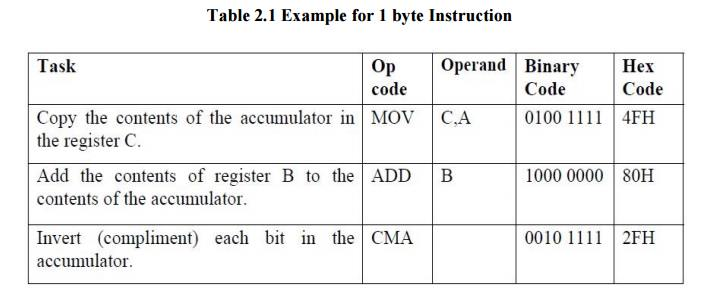Home | | Microprocessors and Microcontrollers | Instruction Format - Programming of 8085 Processor

# Instruction Format - Programming of 8085 Processor

An instruction is a command to the microprocessor to perform a given task on a specified data. Each instruction has two parts: one is task to be performed, called the operation code (opcode), and the second is the data to be operated on, called the operand.

PROGRAMMING OF 8085 PROCESSOR

Instruction Format

An instruction is a command to the microprocessor to perform a given task on a specified data. Each instruction has two parts: one is task to be performed, called the operation code (opcode), and the second is the data to be operated on, called the operand. The operand (or data) can be specified in various ways. It may include 8-bit (or 16-bit) data, an internal register, a memory location, or 8-bit (or 16-bit) address. In some instructions, the operand is implicit.

Instruction word size

The 8085 instruction set is classified into the following three groups according to word size:

ü One-word or 1-byte instructions

ü Two-word or 2-byte instructions

ü Three-word or 3-byte instructions

In the 8085, "byte" and "word" are synonymous because it is an 8-bit microprocessor. However, instructions are commonly referred to in terms of bytes rather than words.

1 One-Byte Instructions

A 1-byte instruction includes the opcode and operand in the same byte. Operand(s) are internal register and are coded into the instructionThese instructions are 1-byte instructions performing three different tasks. In the first instruction, both operand registers are specified. In the second instruction, the operand B is specified and the accumulator is assumed. Similarly, in the third instruction, the accumulator is assumed to be the implicit operand. These instructions are stored in 8- bit binary format in memory; each requires one memory location.

MOV rd, rs

rd ß rs copies contents of rs into rd.

Coded as 01 ddd sss

where ddd is a code for one of the 7 general registers which is the destination of the data, sss is the code of the source register.

Example: MOV A,B

Coded as 01111000 = 78H = 170 octal (octal was used extensively in instruction design of such processors).

A ß A + r

2 Two-Byte Instructions

In a two-byte instruction, the first byte specifies the operation code and the second byte specifies the operand. Source operand is a data byte immediately following the opcode. For example:

Table 2.2 Example for 2 byte InstructionThe instruction would require two memory locations to store in memory.

MVI r,data

r ß data

Example: MVI A,30H coded as 3EH 30H as two contiguous bytes.

This is an example of immediate addressing.

A ß A + data

OUT port

0011 1110

DATA

Where port is an 8-bit device address. (Port) ß A.

Since the byte is not the data but points directly to where it is located this is called direct addressing.

3 Three-Byte Instructions

In a three-byte instruction, the first byte specifies the opcode, and the following two bytes specify the 16-bit address. Note that the second byte is the low-order address and the third byte is the high-order address.

opcode + data byte + data byte

Table 3.3 Example for 3 byte InstructionThis instruction would require three memory locations to store in memory.

Three byte instructions - opcode + data byte + data byte

LXI rp, data16

rp is one of the pairs of registers BC, DE, HL used as 16-bit registers. The two data bytes are 16-bit data in L H order of significance.

rp ß data16

LXI H,0520H coded as 21H 20H 50H in three bytes. This is also immediate addressing.